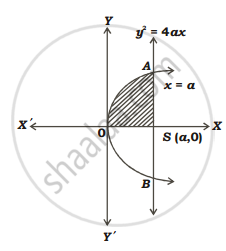HSC Science (General) 12th Board ExamMaharashtra State Board
Share

# Find the area of the region bounded by the parabola y^2 = 4ax and its latus rectum. - HSC Science (General) 12th Board Exam - Mathematics and Statistics

ConceptArea of the Region Bounded by a Curve and a Line

#### Question

Find the area of the region bounded by the parabola y2 = 4ax and its latus rectum.

#### Solution

Given equation of parabola is y2 = 4ax

y=2sqrtasqrtxRequired area = Area of region OBSAO = 2(area of region OSAO)

=2int_0^aydx

=2int_0^a2sqrtasqrtx

=4sqrtaint_0^asqrtxdx

=4sqrta[2/3x^(3/2)]_0^a

=8/3 sqrta[a^(3/2)]

therefore A=8/3a^2 sq.units

Is there an error in this question or solution?

#### Video TutorialsVIEW ALL 

Solution Find the area of the region bounded by the parabola y^2 = 4ax and its latus rectum. Concept: Area of the Region Bounded by a Curve and a Line.
S